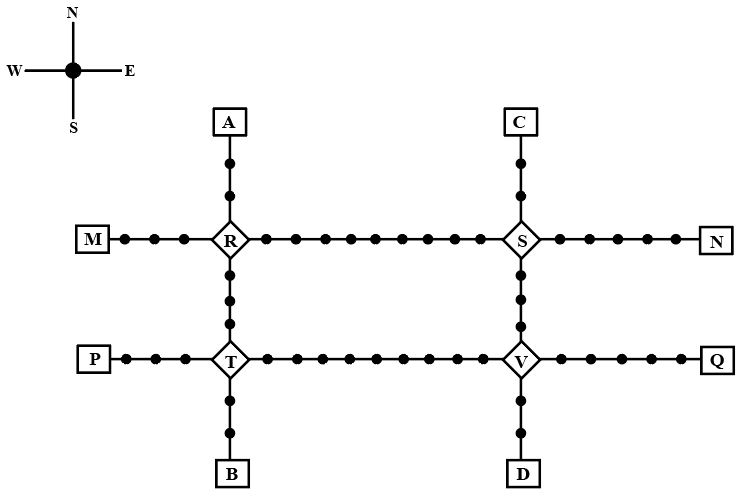### CAT 2022 Question Paper (Slot 1) Question 40

InstructionsGiven above is the schematic map of the metro lines in a city with rectangles denoting terminal stations (e.g. A), diamonds denoting junction stations (e.g. R) and small filled-up circles denoting other stations. Each train runs either in east-west or north-south direction, but not both. All trains stop for 2 minutes at each of the junction stations on the way and for 1 minute at each of the other stations. It takes 2 minutes to reach the next station for trains going in east-west direction and 3 minutes to reach the next station for trains going in northsouth direction. From each terminal station, the first train starts at 6 am; the last trains leave the terminal stations at midnight. Otherwise, during the service hours, there are metro service every 15 minutes in the north-south lines and every 10 minutes in the east-west lines. A train must rest for at least 15 minutes after completing a trip at the terminal station, before it can undertake the next trip in the reverse direction. (All questions are related to this metro service only. Assume that if someone reaches a station exactly at the time a train is supposed to leave, (s)he can catch that train.)

Question 40

# If Hari is ready to board a train at 8:05 am from station M, then when is the earliest that he can reach station N?

Solution

In the east-west direction, a train starts from station M every 10 minutes.

So the earliest by which Hari can catch a train from station M is 8:10 am.

Now there are 19 stations between M and n, out of which two stations are junctions.

Time taken to travel between two stations in the east-west direction is 2 minutes.

Therefore, the time for which the train was running between M and N (excluding the stoppage time) = $$20\times2=40$$ minutes

Stoppage time at a junction is 2 minutes, while at the rest of the stations, it is 1 minute each.

Stoppage time for the train running between M and N = $$\left(17\times1\right)+\left(2\times2\right)=\ 21$$ minutes

Therefore, total travel time = 40+21 = 61 minutes.